# Recursion: Visualizing the ‘sum’ Function

Another way to view recursion is with visual diagrams. To demonstrate this, I’ll use the rectangular symbol shown in Figure [fig:rectFunctionSymbol] to represent a function.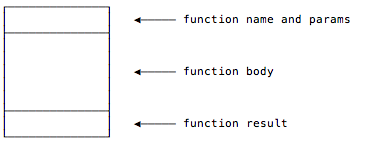Using that symbol and a list with only three elements, Figure [fig:the1stSumCall] shows a representation of the first `sum` function call.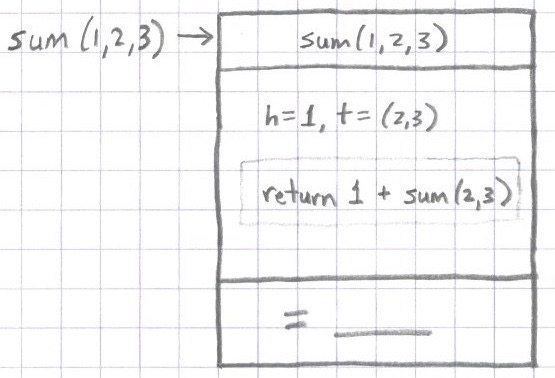The top cell in the rectangle indicates that this first instance of `sum` is called with the parameters `1,2,3`. Note that I’m leaving the “`List`” name off of these diagrams to make them more readable.

The body of the function is shown in the middle region of the symbol, and it’s shown as `return 1 + sum(2,3)`. As I mentioned before, you don’t normally use the `return` keyword with Scala/FP functions, but in this case it makes the diagram more clear.

In the bottom region of the symbol I’ve left room for the final return value of the function. At this time we don’t know what the function will return, so for now I just leave that spot empty.

For the next step of the diagram, assume that the first `sum` function call receives the parameter list `(1,2,3)`, and its body now calls a new instance of `sum` with the input parameter `sum(2,3)` (or `sum(List(2,3))`, if you prefer). You can imagine the second `case` expression separating the `List` into head and tail elements, as shown in Figure [fig:the2ndSumCall].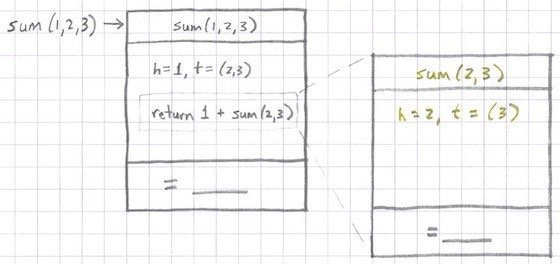Then this `sum` instance makes a recursive call to another `sum` instance, as shown in Figure [fig:the2ndSumCallsThe3rd].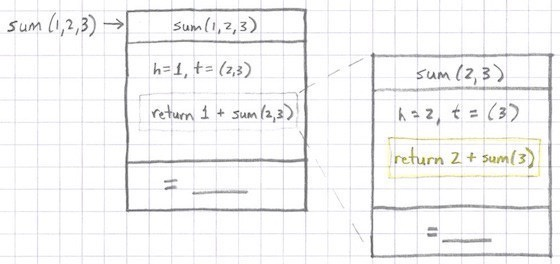Again I leave the return value of this function empty because I don’t know what it will be until its `sum` call returns.

It’s important to be clear that these two function calls are completely different instances of `sum`. They have their own input parameter lists, local variables, and return values. It’s just as if you had two different functions, one named `sum3elements` and one named `sum2elements`, as shown in Figure [fig:justLikeCallingDiffFunction].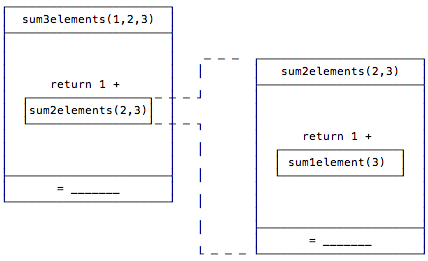Just as the variables inside of `sum3elements` and `sum2elements` have completely different scope, the variables in two different instances of `sum` also have completely different scope.

Getting back to the `sum` example, you can now imagine that the next step will proceed just like the previous one, as shown in Figure [fig:the3rdSumCall].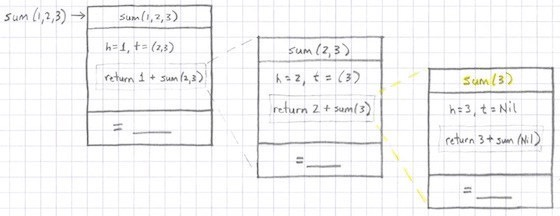Now we’re at the point where we make the last recursive call to `sum`. In this case, because `3` was the last integer in the list, a new instance of `sum` is called with the `Nil` value. This is shown in Figure [fig:nilPassedIntoLastSumCall].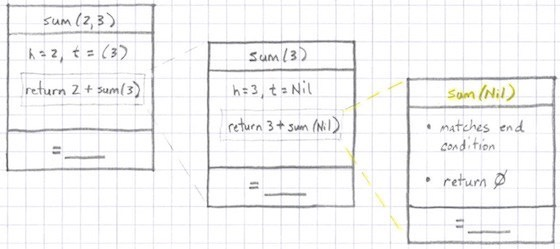With this last `sum` call, the `Nil` input parameter matches the first `case` expression, and that expression simply returns `0`. So now we can fill in the return value for this function, as shown in Figure [fig:returnValLastCallIs0].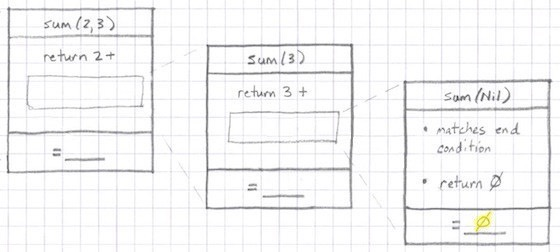Now this `sum` instance returns `0` back to the previous `sum` instance, as shown in Figure [fig:return0To3rdSumCall].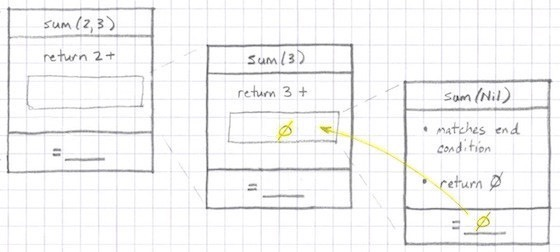The result of this function call is `3 + 0` (which is `3`), so you can fill in its return value, and then flow it back to the previous `sum` call. This is shown in Figure [fig:the3rdSumCallReturns].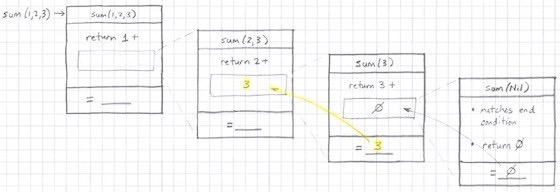The result of this function call is `2 + 3` (`5`), so that result can flow back to the previous function call, as shown in Figure [fig:the2ndSumCallReturns].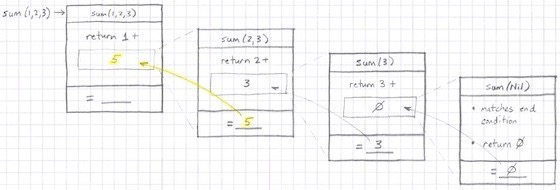Finally, the result of this `sum` instance is `1 + 5` (`6`). This was the first `sum` function call, so it returns the value `6` back to whoever called it, as shown in Figure [fig:the1stSumCallReturns].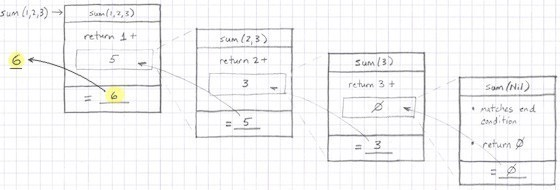There are other ways to draw recursive function calls. Another nice approach is to use a modified version of a UML “Sequence Diagram,” as shown in Figure [fig:sumFunctionCallsAsSequenceDiagram]. Note that in this diagram “time” flows from the top to the bottom.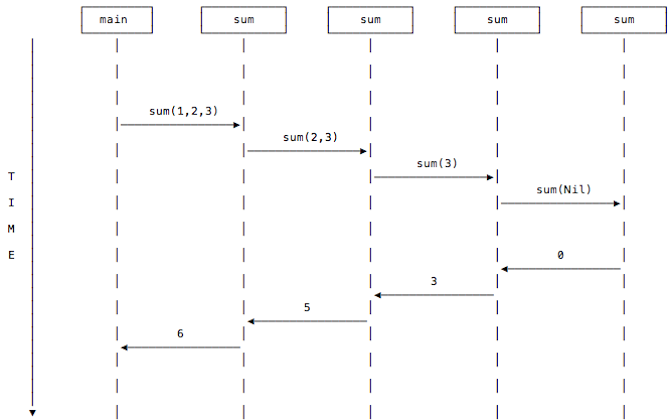This diagram shows that the `main` method calls `sum` with the parameter `List(1,2,3)`, where I again leave off the `List` part; it calls `sum(2,3)`, and so on, until the `Nil` case is reached, at which point the return values flow back from right to left, eventually returning `6` back to the `main` method.

You can write the return values like that, or with some form of the function’s equation, as shown in Figure [fig:writingFunctionReturnValuesAsEquations].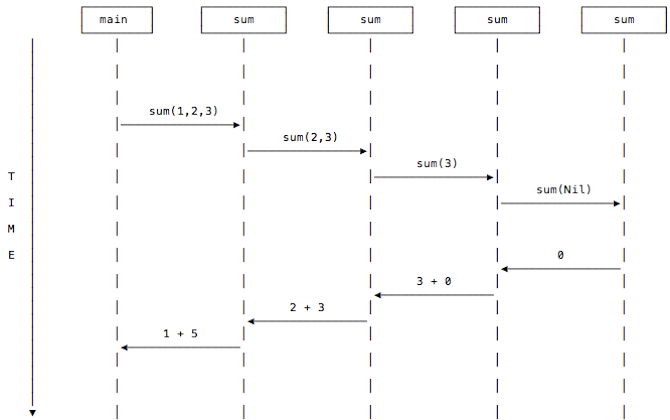Personally, I use whatever diagram seems to help the most.

Those are some visual examples of how recursive function calls work. If you find yourself struggling to understand how recursion works, I hope these diagrams are helpful.